Hostname: page-component-7d684dbfc8-8ckrc Total loading time: 0 Render date: 2023-09-23T05:33:28.569Z Has data issue: false Feature Flags: { "corePageComponentGetUserInfoFromSharedSession": true, "coreDisableEcommerce": false, "coreDisableSocialShare": false, "coreDisableEcommerceForArticlePurchase": false, "coreDisableEcommerceForBookPurchase": false, "coreDisableEcommerceForElementPurchase": false, "coreUseNewShare": true, "useRatesEcommerce": true } hasContentIssue false

# A DEFINABLE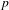$p$-ADIC ANALOGUE OF KIRSZBRAUN’S THEOREM ON EXTENSIONS OF LIPSCHITZ MAPS

Published online by Cambridge University Press:  20 October 2015

## Abstract

A direct application of Zorn’s lemma gives that every Lipschitz map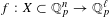$f:X\subset \mathbb{Q}_{p}^{n}\rightarrow \mathbb{Q}_{p}^{\ell }$ has an extension to a Lipschitz map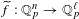$\widetilde{f}:\mathbb{Q}_{p}^{n}\rightarrow \mathbb{Q}_{p}^{\ell }$. This is analogous to, but easier than, Kirszbraun’s theorem about the existence of Lipschitz extensions of Lipschitz maps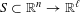$S\subset \mathbb{R}^{n}\rightarrow \mathbb{R}^{\ell }$. Recently, Fischer and Aschenbrenner obtained a definable version of Kirszbraun’s theorem. In this paper, we prove in the$p$-adic context that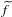$\widetilde{f}$ can be taken definable when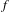$f$ is definable, where definable means semi-algebraic or subanalytic (or some intermediary notion). We proceed by proving the existence of definable Lipschitz retractions of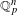$\mathbb{Q}_{p}^{n}$ to the topological closure of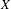$X$ when$X$ is definable.

Type
Research Article
Information

## Access options

Get access to the full version of this content by using one of the access options below. (Log in options will check for institutional or personal access. Content may require purchase if you do not have access.)

## References

Aschenbrenner, M. and Fischer, A., Definable versions of theorems by Kirszbraun and Helly, Proc. Lond. Math. Soc. (3) 102(3) (2011), 468502.CrossRefGoogle Scholar
Bhaskaran, R., The structure of ideals in the Banach algebra of Lipschitz functions over valued fields, Compos. Math. 48(1) (1983), 2534.Google Scholar
Cluckers, R., Presburger sets and p-minimal fields, J. Symbolic Logic 68 (2003), 153162.CrossRefGoogle Scholar
Cluckers, R., Comte, G. and Loeser, F., Lipschitz continuity properties for p-adic semi-algebraic and subanalytic functions, Geom. Funct. Anal. 20(1) (2010), 6887.CrossRefGoogle Scholar
Cluckers, R. and Halupczok, I., Approximations and Lipschitz continuity in p-adic semi-algebraic and subanalytic geometry, Selecta Math. (N.S.) 18(4) (2012), 825837.CrossRefGoogle Scholar
Cluckers, R. and Lipshitz, L., Fields with analytic structure, J. Eur. Math. Soc. (JEMS) 13(4) (2011), 11471223.CrossRefGoogle Scholar
Cohen, P. J., Decision procedures for real and p-adic fields, Comm. Pure Appl. Math. 22 (1969), 131151.CrossRefGoogle Scholar
Darnière, L. and Halupczok, I., Cell decomposition and classification of definable sets in$p$ -optimal fields (2015) arXiv:1412.2571.Google Scholar
Denef, J., The rationality of the Poincaré series associated to the p-adic points on a variety, Invent. Math. 77 (1984), 123.CrossRefGoogle Scholar
Denef, J., p-adic semialgebraic sets and cell decomposition, J. Reine Angew. Math. 369 (1986), 154166.Google Scholar
Denef, J. and van den Dries, L., p-adic and real subanalytic sets, Ann. of Math. (2) 128(1) (1988), 79138.CrossRefGoogle Scholar
van den Dries, L., Algebraic theories with definable Skolem functions, J. Symbolic Logic 49(2) (1984), 625629.CrossRefGoogle Scholar
Halupczok, I., Trees of definable sets over the p-adics, J. Reine Angew. Math. 642 (2010), 157196.Google Scholar
Halupczok, I., Trees of definable sets in ℤ p , in Motivic Integration and its Interactions with Model Theory and Non-Archimedean Geometry, Vol. II, London Mathematical Society Lecture Note Series, 384, pp. 87107 (Cambridge University Press, Cambridge, 2011).CrossRefGoogle Scholar
Haskell, D. and Macpherson, D., A version of o-minimality for the p-adics, J. Symbolic Logic 62(4) (1997), 10751092.CrossRefGoogle Scholar
Kirszbraun, M. D., Über die zusammenziehende und Lipschitzsche Transformationen, Fund. Math. 22 (1934), 77108.Google Scholar
Kuijpers, T., Lipschitz extensions of definable p-adic functions, Math. Log. Q. 61(3) (2015), 151158.CrossRefGoogle Scholar
Macintyre, A., On definable subsets of p-adic fields, J. Symbolic Logic 41 (1976), 605610.CrossRefGoogle Scholar
Margalef Roig, J. and Outerelo Domínguez, E., Differential Topology, North-Holland Mathematics Studies, 173, (North-Holland Publishing Co., Amsterdam, 1992). With a preface by P. W. Michor.Google Scholar
McShane, E. J., Extension of range of functions, Bull. Amer. Math. Soc. 40 (1934), 837842.CrossRefGoogle Scholar
Pas, J., Cell decomposition and local zeta functions in a tower of unramified extensions of a p-adic field, Proc. Lond. Math. Soc. (3) 60(1) (1990), 3767.CrossRefGoogle Scholar
Presburger, M., On the completeness of a certain system of arithmetic of whole numbers in which addition occurs as the only operation, Hist. Philos. Logic 12(2) (1991), 225233.CrossRefGoogle Scholar
Whitney, H., Analytic extensions of functions defined in closed sets, Trans. Amer. Math. Soc. 36 (1934), 6389.CrossRefGoogle Scholar